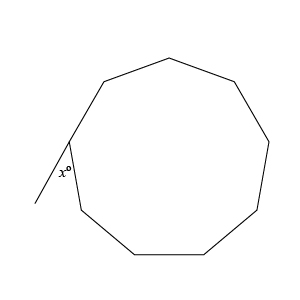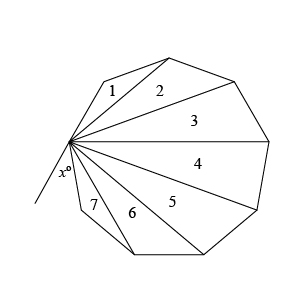# The figure above shows a regular 9-sided polygon. What is the valueThe figure above shows a regular 9-sided polygon. What is the value of \$x\$ ?

So, you were trying to be a good test taker and practice for the GRE with PowerPrep online. Buuuut then you had some questions about the quant section—specifically question 13 of the second Quantitative section of Practice Test 1. Those questions testing our knowledge of Polygons can be kind of tricky, but never fear, PrepScholar has got your back!

## Survey the Question

Let’s search the problem for clues as to what it will be testing, as this will help shift our minds to think about what type of math knowledge we’ll use to solve this question. Pay attention to any words that sound math-specific and anything special about what the numbers look like, and mark them on our paper.

Let’s keep what we’ve learned about this skill at the tip of our minds as we approach this question.

## What Do We Know?

Let’s carefully read through the question and make a list of the things that we know.

1. We have a regular \$9\$-sided polygon
2. We want to know the value of an external angle to that polygon shown in the figure

## Develop a Plan

We know that the sum of angles on one side of a straight line is \$180°\$ from the figure, we can see that if we can find the value of the interior angle at one vertex of the polygon, then we can subtract that value from \$180°\$ to get the value of \$x\$.

To find the interior angle of any polygon, we can divide it into triangles, knowing that all triangles have internal angles that sum up to \$180°\$. Then multiply the number of triangles by \$180°\$ and finally divide by the number of vertices of the polygon to get the value of its interior angle. This won’t be as difficult as it sounds, particularly once we start drawing the triangles on our figure.

## Solve the Question

First, let’s draw triangles starting at one vertex in our figure, like this:So here we can see that the sum of all of the internal angles in our polygon can be represented as seven triangles. To find the value of an internal angle within this polygon, we can just multiply the number of triangles by \$180°\$, then divide by the number of internal angles, which is nine.

 \$\Interior \Angle \of \a \Polygon\$ \$=\$ \${180°·\Number \of \Triangles}/{\Number \of \Vertices}\$ \$ \$ \$ \$ \$\Interior \Angle \of \a \Polygon\$ \$=\$ \${180°·7}/9\$ \$ \$ \$ \$ \$\Interior \Angle \of \a \Polygon\$ \$=\$ \${9·20°·7}/9\$ \$ \$ \$ \$ \$\Interior \Angle \of \a \Polygon\$ \$=\$ \$20°·7\$ \$ \$ \$ \$ \$\Interior \Angle \of \a \Polygon\$ \$=\$ \$140°\$

Excellent! So the interior angle of a \$9\$-sided polygon is \$140°\$. We can see that \$x\$ and one interior angle lie on the same side of a straight line, so their sum must be \$180°\$. So \$x=180°-140°\$, or \$x=40°\$.